Share

Books Shortlist

# Textbook Solutions for CBSE (Science) Class 12 - CBSE

< prev 1 to 6 of 6 next >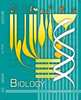NCERT Solutions for Class 12 Biology Textbook Biology

Chapters : Reproduction in Organisms, Sexual Reproduction in Flowering Plants, Human Reproduction, Reproductive Health, Principles of Inheritance and Variation, Molecular Basis of Inheritance, Evolution, Human Health and Disease, Strategies for Enhancement in Food Production, Microbes in Human Welfare, Biotechnology : Principles and Processes, Biotechnology and its Applications, Organisms and Populations, Ecosystem, Biodiversity and Conservation, Environmental Issues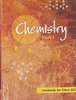NCERT Solutions for Class 12 Chemistry Textbook Chemistry

Chapters : The Solid State, Solutions, Electrochemistry, Chemical Kinetics, Surface Chemistry, General Principles and Processes of Isolation of Elements, The p-block Elements, The d-block and f-block Elements, Coordinate Compounds, Haloalkanes and Haloarenes, Alcohols, Phenols and Ethers, Aldehydes, Ketones and Carboxylic Acids, Amines, Biomolecules, Polymers, Chemistry in Everyday Life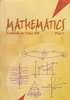NCERT Solutions for Class 12 Mathematics Textbook Mathematics

Chapters : Relations and Functions, Inverse Trigonometric Functions, Matrices, Determinants, Continuity and Differentiability, Application of Derivatives, Integrals, Application of Integrals, Differential Equations, Vector Algebra, Three Dimensional Geometry, Linear Programming, Probability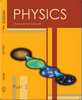NCERT Solutions for Class 12 Physics Textbook Physics

Chapters : Electric Charge and Fields, Electrostatic Potential and Capacitance, Current Electricity, Moving Charges and Magnetism, Magnetism and Matter, Electromagnetic Induction, Alternating Current, Electromagnetic Waves, Ray Optics and Optical Instruments, Wave Optics, Dual Nature of Radiation and Matter, Atoms, Nuclei, Semiconductor Electronics: Materials, Devices and Simple Circuits, Communication Systems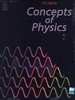H.C. Verma Solutions for Concept of Physics 1 by H.C Verma Physics

Chapters : Introduction to Physics, Physics and Mathematics, Rest and Motion: Kinematics, The Forces, Newton's Laws of Motion, Friction, Circular Motion, Work and Energy, Centre of Mass, Linear Momentum, Collision, Rotational Mechanics, Gravitation, Simple Harmonics Motion, Fluid Mechanics, Some Mechanical Properties of Matter, Wave Motion and Waves on a String, Sound Waves, Light Waves, Geometrical Optics, Optical Instruments, Dispersion and Spectra, Speed of Light, Photometry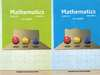RD Sharma Solutions for Mathematics for Class 12 by R D Sharma Mathematics

Chapters : Relations, Functions, Binary Operations, Inverse Trigonometric Functions, Algebra of Matrices, Determinants, Adjoint and Inverse of a Matrix, Solution of Simultaneous Linear Equations, Continuity, Differentiability, Differentiation, Higher Order Derivatives, Derivative as a Rate Measurer, Differentials, Errors and Approximations, Mean Value Theorems, Tangents and Normals, Increasing and Decreasing Functions, Maxima and Minima, Indefinite Integrals, Definite Integrals, Areas of Bounded Regions, Differential Equations, Algebra of Vectors, Scalar Or Dot Product, Vector or Cross Product, Scalar Triple Product, Direction Cosines and Direction Ratios, Straight Line in Space, The Plane, Linear programming, Probability, Mean and Variance of a Random Variable, Binomial Distribution

< prev 1 to 6 of 6 next >

## Textbook solutions and Reference book solutions for Class 12

• On this page you will find NCERT solution, H.C. Verma solution, RD Sharma solution for Class 12.
• Each chapter comes with subsections that provide in-depth knowledge about the basic concepts.
• Solved equations along with examples help the students to understand the different steps towards solving their exercises.
• Compilation of different exercises makes it a great choice for students to practice to brush up their concepts and skills.
• These books are a must-have for the students who want to closely evaluate the different aspects of their respective subjects.
• Different graphs along with schematic representations help the students to memorise the many steps.
• These solutions for Class 12 are prepared by experts, include the problems from latest edition and are as per CBSE pattern.

## Reference book solutions and textbook solutions on Shaalaa.com

Shaalaa.com is a great source for Reference book solutions and textbook solutions of all Subjects for Practice. Our best teachers have reviewed all the Class 12 solutions of exercise questions that will help you solve all the Class 12 questions of all subject without any problem. Reference book Solutions are highly beneficial especially when you are thinking to prepare for school as well as competitive level examinations. We at Shaalaa.com work hard to create better ways in order to help students and provide you with the detailed solutions for all questions from reference boook and textbook as per the latest marking scheme pattern is to back you up in effective learning in all the examinations conducted by CBSE.
S